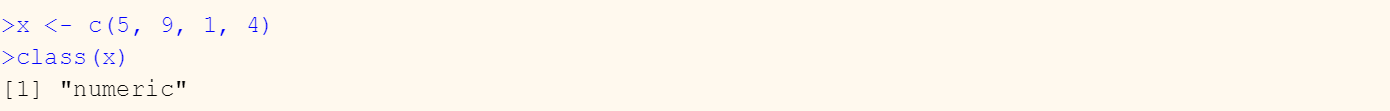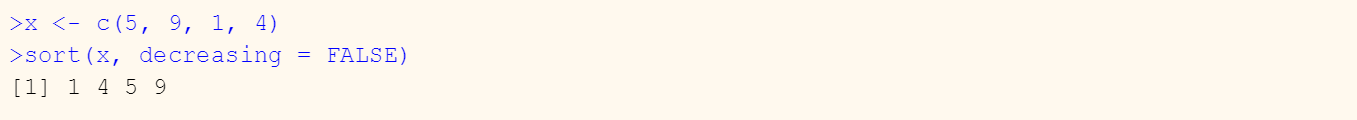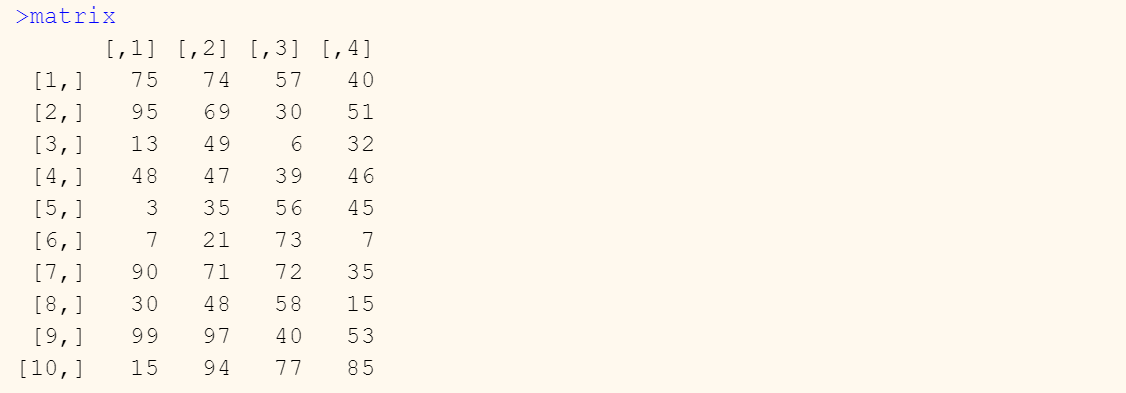Want to share your content on R-bloggers? click here if you have a blog, or here if you don't.

There are several different methods for sorting data in R. The best method depends on on the type of data structure you have. In R, you can store data in different object types such as vectors, data frames, matrices and arrays. There are a range of other more complex structures in R, but we will just cover sort functions for some of the more common data types.

## Object Classes

You can identify the type of data structure being used with the class() function, which will return the data type of the object. In the example below, we see that x is a numeric vector of values.## Sorting Vectors

In R, a vector is one-dimensional lists of values of the same basic data type, such as text or numeric. A simple vector containing 4 numeric values may look like this:To sort a vector in R use the sort() function. See the following example.By default, R will sort the vector in ascending order. However, you can add the decreasing argument to the function, which will explicitly specify the sort order as in the example above.

## Sorting Data Frames

In R, a data frame is an object with multiple rows and multiple columns. Each column in a data frame can be of a different data type. To sort data frames, use the order() function. Consider the following R data frame (df) which contains data on store location, account rep, number of employees and monthly sales:To sort the data frame in descending order by monthly sales, apply the order function with the column to sort by specified in the function:Note that the negative sign (-) in front of the column name (df\$sales) is applied to execute the sort in descending order. You can also use the decreasing argument, as in the sort() function.

The order() function can also reference the column index rather than the specific column name. For example, the same sort can be achieved using the following syntax to reference the fourth column in the data frame:You can also sort by multiple columns by specifying multiple arguments in the sort function. For example, suppose we wanted to first sort the above data frame by sales rep as the primary sort in ascending order and then by monthly sales in descending order.## Sorting Matrices

A matrix is similar to a data frame except in that all columns in a matrix must be of the same data type (numeric, character, etc.). Consider the following 4×10 matrix of numeric values.To sort the matrix by the first column in ascending order, we would use the same sort function that we used to previously sort a data frame:Note that we are referencing the first column in the sort function. You can also sort by adding additional column references to the order function. For example, to sort the above matrix by the first column in ascending order as the primary sort and the second column as the secondary sort, add a second column reference to the order function. Note the negative (-) sign in front of the second sort term. This sorts the second column in descending order.We hope you found this post helpful. Find out how to do more in R by checking out our “How to do this in R” series!